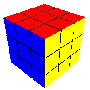# Puzzle of the Week for 10 May 1999How mixed up can a Rubik's cube become? Scramble a Rubik's cube, look at each pair of adjacent faces, and count the number of squares of each color. The largest number you obtain (let's call it S) is a measure of order. Since there are 9 squares of each color, the highest possible value of S is 9. What is the lowest possible value of S, corresponding to the most scrambled arrangement?